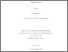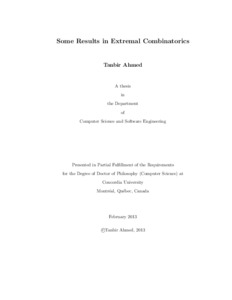Title:

# Some Results in Extremal Combinatorics

Ahmed, Tanbir (2013) Some Results in Extremal Combinatorics. PhD thesis, Concordia University.Preview Text (application/pdf) Ahmed_PhD_S2103.pdf - Accepted Version Available under License Spectrum Terms of Access. 537kB

## Abstract

Extremal Combinatorics is one of the central and heavily contributed areas in discrete mathematics,
and has seen an outstanding growth during the last few decades. In general, it deals
with problems regarding determination and/or estimation of the maximum or the minimum size
of a combinatorial structure that satisfies a certain combinatorial property. Problems in Extremal
Combinatorics are often related to theoretical computer science, number theory, geometry, and information
theory. In this thesis, we work on some well-known problems (and on their variants) in
Extremal Combinatorics concerning the set of integers as the combinatorial structure.

The van der Waerden number w(k;t_0,t_1,...,t_{k-1}) is the smallest positive
integer n such that every k-colouring of 1, 2, . . . , n contains a monochromatic
arithmetic progression of length t_j for some colour j in {0,1,...,k-1}. We have
determined five new exact values with k=2 and conjectured several van der Waerden numbers
of the form w(2;s,t), based on which we have formulated a polynomial
upper-bound-conjecture of w(2; s, t) with fixed s. We have provided an efficient SAT
encoding for van der Waerden numbers with k>=3 and computed three new van der Waerden
numbers using that encoding. We have also devised an efficient problem-specific
backtracking algorithm and computed twenty-five new van der Waerden numbers with k>=3
using that algorithm.

We have proven some counting properties of arithmetic progressions and some unimodality
properties of sequences regarding arithmetic progressions. We have generalized Szekeres’
conjecture on the size of the largest sub-sequence of 1, 2, . . . , n without an
arithmetic progression of length k for specific k and n; and provided a construction for
the lower bound corresponding to the generalized conjecture.

A Strict Schur number S(h,k) is the smallest positive integer n such that every
2-colouring of 1,2,...,n has either a blue solution to x_1 +x_2 +···+x_{h-1} = x_h
where x_1 < x_2 < ··· < x_h, or a red solution to x_1+x_2+···+x_{k-1} =x_k where
x_1 <x_2 <···<x_k. We have proven the exact formula for S(3, k).

Divisions: Concordia University > Gina Cody School of Engineering and Computer Science > Computer Science and Software Engineering Thesis (PhD) Ahmed, Tanbir Concordia University Ph. D. Computer Science 8 February 2013 Lam, Clement Extremal Combinatorics, Van der Waerden numbers, Strict Schur Numbers, Arithmetic Progressions, Sets, Sequences 976929 TANBIR AHMED 17 Jun 2013 15:42 18 Jan 2018 17:43
All items in Spectrum are protected by copyright, with all rights reserved. The use of items is governed by Spectrum's terms of access.

Repository Staff Only: item control pageResearch related to the current document (at the CORE website)
Back to top